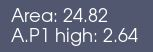# Measure exact the alpha.phase 1 level

Hey Forum,
is it possible to measure automatically the distance from the bottom to the alpha.phase 1 level end ?
I want to get the relationship between h (heigt) and Q (flux), h(Q), therefor i need a good measurment of the waterlevel.
Below is a picture of my system.

Its a very coarse Mesh, it will get finer. My idea is, to sum up the cell high where the alpha.phase1 >= 0.8 for example, is it possible ?

Best regards
Kai

Sure, you can sum up the cell volume where alpha.phase1 >-= 0.8. You can use a Python Annotation filter and set the Expression to something like

`sum(volume(inputs) * (alpha.phase1 >= 0.8))`

`volume` computes the cell volumes for each cell, `alpha.phase1 >= 0.8` produces a 1 where the threshold criterion is met and 0 otherwise, and `sum` adds up all the cell volumes.

Dividing the volume by the surface area should give you the height.

1 Like

@cory.quammen,
With this expression i get the volume where the AP.1 is over or similar to 0.8.

``````sum(volume(inputs) * (alphaphase1 >= 0.8))
``````

if i divide it with the width and length, i get the high.

``````sum(volume(inputs) * (alphaphase1 >= 0.8))/(5*5)
``````

But if i dont know the groundarea, its possbile to calculate this too ?

And a little offtopic question. I marked one cell at the front surface, can i get a few information about the marked cell e. g. length in x, y, and z direction ?

aunt edit:
I think i finde a way to display the cell dimensions. I extract the selection (one Cell) and then the information is displayed.

Maybe there is a way with less mouseclicksbest regards
Kai

Sure. You can first use Extract Surface filter to get just the surface of your domain. From that select the cells representing the ground using the cell selection tools (the tiny icons above the render view). Finally, extract those cells with an Extract Selection filter, then add a Python Annotation with the expression `sum(area(inputs))`.

Nope, that’s about the easiest way.

1 Like

Thank you very much !

``````"Area: " + format(sum(area(inputs)), '.2f')
``````

and
`"A.P1 high: " + format(sum(volume(inputs) * (alphaphase1 >= 0.8)/ 24.82),'.2f')`If i may ask one more question, is it possible to assign the area to a variable like
`A = sum(area(inputs))`

Thank you very much @cory.quammen

Instead of Python Annotation only, you can use Programmable Filter to compute both volume and surface area from the full and extracted dataset.

First, click the original dataset in the Pipeline Browser, then Ctrl-click the Extract Surface Filter, so you have two selected filters in the browser. Now add a Programmable Filter with the following script:

``````from paraview import vtk

height = sum(volume(inputs) * (alphaphase1 >= 0.8)) / sum(area(inputs))
output.FieldData.append(height, "height")
``````

Finally, add a Python Annotation with Array Association set to “Field Data” and Expression set to `height`.

1 Like

Thank you very much @cory.quammen

Best regards
Kai

I’m not getting this with the Programmable Filter

The pipline browser looks like this:

With an ExtractSelection at the bottom, and a blue rectangle.
I write your script in the ProgrammableFilter, but i got this error message:

``````NameError: global name 'alphaphase1' is not defined
Traceback (most recent call last):
File "<stron>", line 24, in <modul>
File"<strin>" line 4, un RequestData
``````

if i tried the script, without the `* (alphaphase1 >= 0.8)`, i got no error at this point.
Then the error comes along with the PythonAnnotation, i got a hugh error message:

best regards
Kai

From the Pipeline Browser, it looks like one input is the Extract Selection filter and the other input is Extract Surface. That second input should be the Clip4 filter instead of Extract Surface, assuming that is the volume you want to process.

1 Like

I’m really sorry I can’t do this.I think i choose the right filter in the pipeline browser.

Clip was choosen.

ExtractSelection was choosen.

MAy should I just send you my openFoam simulation? And you look into it ?

Best regards
Kai

Hey @cory.quammen,